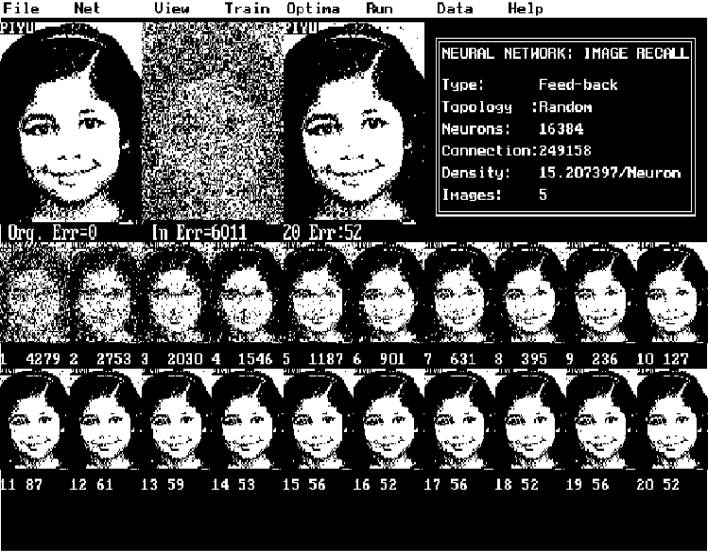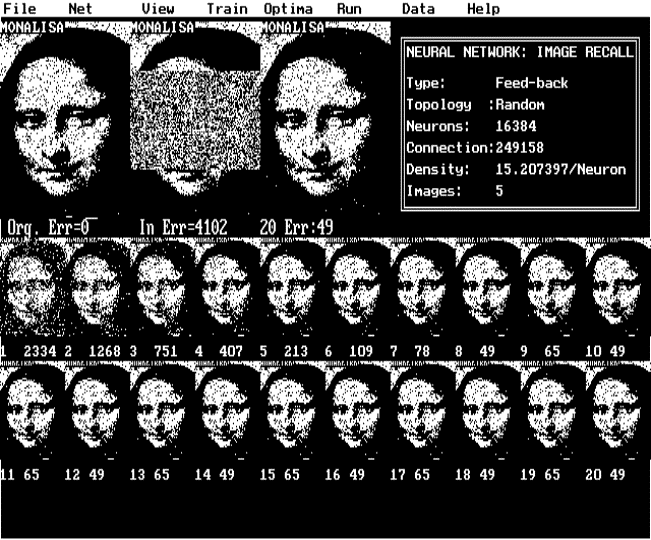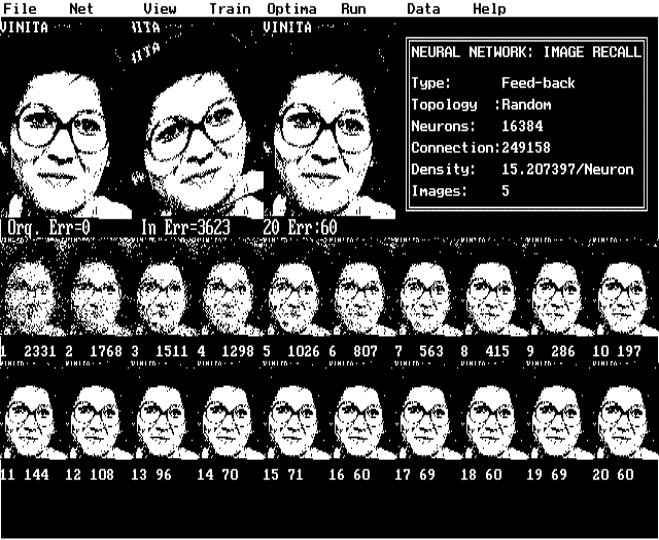RENT A THINKER Home My Page Chat Tik-Tok
9        RANDOMLY CONNECT FEED-BACK TYPE NEURAL NETWORK FOR ASSOCIATIVE IMAGE MEMORY

9.6       References

9.7        Publication

### 9.1       Abstract

Our brain has excellent associative image memory. We do not understand it fully well as how brain stores and recalls images.  It is unlikely that natural neural circuits in our brain are fully and orderly connected like Hopfield type network. Neurons are partially and randomly interconnected in our brain. It is interesting to study the topology of such networks and there properties. We will discuss about the techniques of building partially randomly connected feedback type networks for associative memory applications. We will also examine the methods of training and optimization of such networks.

### 9.2       Introduction

Hopfield type neural networks are used as associative memory and for multi-variable optimization problems. In Hopfield type network, generally binary output is used for each neuron. Generally the output levels used are  -1 or +1. Inputs of every neuron are connected through network elements to all other neurons' output through connections having appropriate weights. The input of a neuron is computed by summing the of products of the output of all other neurons and there corresponding connection weights to the given neuron as shown in equation-1. The output of a neuron is updated using equation-2, by hard clipping the sum of input values. Generally the output is +1 or -1 depending on the sign of the input. To store a set of patterns in these network the connection weights are fixed using Hebb’s rule [equation (3)].

Hopfield's network-

N-1
Yj(t+1) = fn
å [  Wij * Yi(t) ]     for (i <> j)   .......(1)

i=0

fn[x] = -1      for x < 0

=  1      for x >= 0                               .......(2)

Where  Yj(t+1) is updated state of the neuron,

Yi(t) is earlier state of the neuron,

Wij is the connection strength between

output of Yi and input of Yj,

N is the total number of neurons.

Assigning of weights-

M-1
Wij =
å  Yi(s)*Yj(s)                           .......(3)

0
where i <> j,

Wij is the connection strength between  input of Yj and output of Yi,

M is number of patterns and,

for i=j, Wij=o.

These networks exhibit the property of recalling the originally trained image from a partial or a noisy input pattern. The interconnection requirements for such a fully connected network is [ N ( N - 1) ], where N is the number of neurons in the network.   A Hopfield type neural network is often used for imaging applications, in which each neuron represents a pixel. However in Hopfield model, number of interconnections increases exponentially with increases of neurons.   Number of interconnections required for a picture having 128 X 128 (16K) pixels is approximately 250 million. The time taken to compute the stable state of the network output is very high for any practical application. The number of patterns that could be stored in a Hopfield type network is approximately 0.15 times the total number of neurons in the network      Brain has excellent associative image memory and yet we do not understand fully well the mechanism of storing and recalling used by the brain.     It is unlikely that natural neural circuit in our brain is fully and orderly connected like Hopfield network. It is connected randomly with a constrain of geometric weighting function deciding the interconnection density. Connection probabilities with neighboring neurons are higher than the ones at a distance.

The work done in this area is at a very primitive stage. One of the reasons for this is the high computational requirement. Even to experiment with a low quality binary image we need very large memory and CPU time.

In view of the above a randomly connected feedback type network model is developed using limited interconnections. Using this method a factor of thousand memory saving is possible with equivalent increase in speed . A prototype network is designed for 128X128 binary picture containing N=16K neurons. Each neuron input has only few (C = 7 to 40) network elements connected to the outputs of randomly selected neurons. 250 thousand interconnections are used instead of 250 million connections required for fully connected network. This gives an  advantage of  3 orders of magnitude in computation time and memory requirement. By connecting these elements homogeneously but randomly between the neurons, we have [ (100 X C)/N % ] 0.1%  probability (for C=16) of any two neurons being  connected in a positive feedback loop. To increase this probability various random network topologies are developed for different types of noise  present in the recall pattern. The Hebb's rule was found inadequate for optimally storing the images in above type of network. An iterative training strategy is developed that converges the output error to less than 0.01% to 0.1%. For efficient computation, a topological mapping function is generated for each connecting element. The network topology is defined in a separate mapping file, which is used, for computation of 'recalls' and  'training' algorithms. A network of size 128 x 128 neurons and 7 links per neurons is used to train seven binary images. Interconnection weights are first computed and then adopted by applying iterative correction proportional to the output error. After the training, the network is tested with any one of the seriously degraded, rotated or shifted images.  Putting random value to randomly chosen pixels or continuous cluster of pixels performs the pixel degradation. Alternately the input image is rotated by a small angle or shifted in both x and y coordinates before presenting to the network (see fig-2).

### 9.3       Network Topology

One of the network topologies developed that gave optimal performance is designed to divide the association into two groups  (I) for near and (II) for far neurons. Part of the interconnections is distributed to adjacent neurons and the other part of the interconnections is distributed over the rest of the neurons. While doing this the probability of positive feedback (i.e. two neurons connected back to back) is increased using external constrain.

### 9.4       Training Algorithm

To optimize the training time the network elements are computed using Hebb's rule [equation (3)]. A bias link is added to each neuron that is found to reduce the error in iterative training mode. The network is trained by computing the analog error with respect to a dynamic threshold value.  The link weight correction is applied   proportional to the  analog error. Figure (2) shows the error convergence curve with and without Hebbian rule applied.

### 9.5       Results

Table (1) Number of images successfully recalled v/s number of elements per neuron.Figure (1) The input and output with randomly distributed noise.Figure (2) The input output with 75% masking on the image.Figure (3) Rotted image response.

### 9.6       References

 Lippmann R.P.,  An introduction to computing with Neural Nets. IEEE ASSP Magazine, pp 4-22, 1987.

 Griff Bilbro etal, Optimization by Mean Field Annealing Advances in Neural Information Processing Systems I.

 Robert J. Marks II etal, Alternting Projection Neural Networks. IEEE transations on circuits and systems, Vol. 36, No. 6, JUNE 1989.

 Nikitas J. Dimopoulos, A Study of the Asympotic Behavior of Neural Networks. IEEE transations on circuits and systems, Vol. 36, No. 5, MAY 1989.